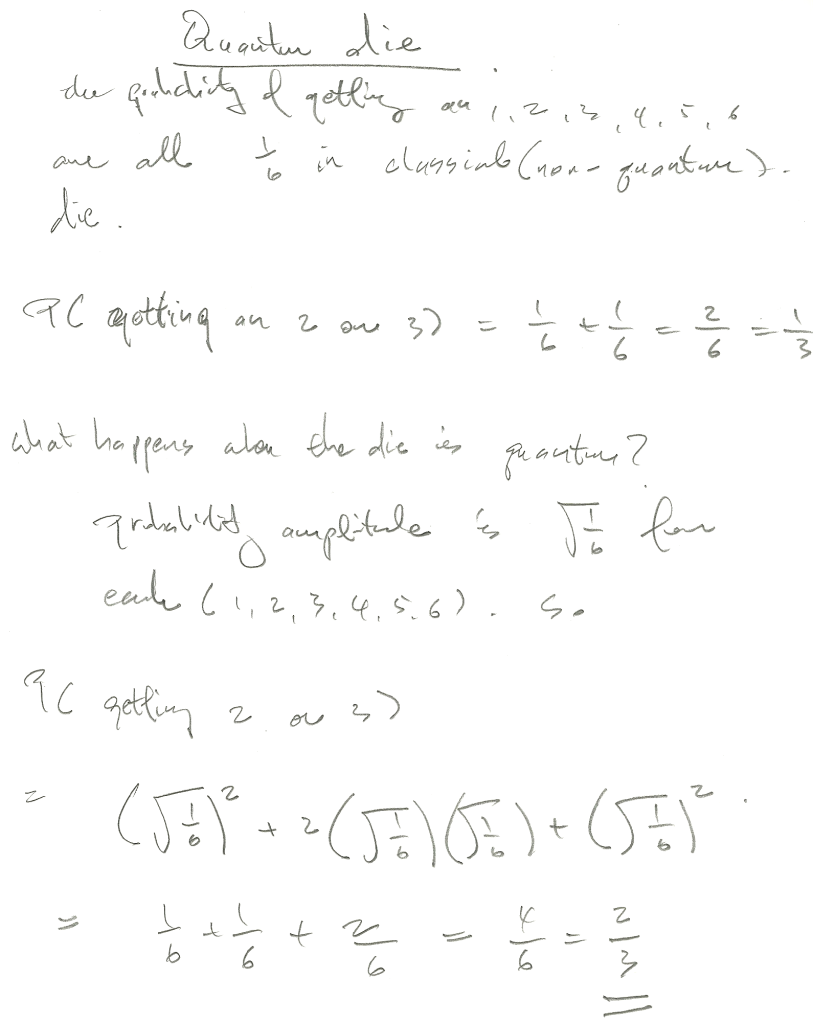# De re probability, a case study from quantum probability

1. Philosophy Forum
2. » Metaphysics
3. » De re probability, a case study from quantum probability

Wed 28 Apr, 2010 01:45 am
A lot of people think probability express once` s degree of belief. I think this might not be the case. Here is why: Imagine an original die( 6 sides). the probability of getting a 3, or 4 is 1/3. Now, imagine a new die that is a quantum object( again, 6 sides). What is the probability of getting a 3, or 4? It is actually 2/3.

Why? See: http://www.philosophyforum.com/blogs/turingequivalent/946-probability-quantum-mechanics-weird.html

This example seems to show a marked difference between a ordinary die, and a quantum die. Probability no looking seem to express our degree of belief, but rather a property of the object in question. The quantum die has the property of obeying some weird addition rules for probability than normal objects.

TuringEquivalent

Fri 30 Apr, 2010 12:38 am
@TuringEquivalent,prothero

Fri 30 Apr, 2010 01:28 am
@TuringEquivalent,
What ever do you mean?
What has probability to do with belief?
Quantum probabilities are stochastic not random.
Quite different from rolling a dice. On a dice all possibilities are equally probable for each event, no so for quantum wave equations where some events (locations, particles)are much more probable than others at each event (measurement).

TuringEquivalent

Fri 30 Apr, 2010 01:33 am
@prothero,
prothero;158293 wrote:

1 What ever do you mean?

2 What has probability to do with belief?

3 Quantum probabilities are stochastic not random.

4 Quite different from rolling a dice. On a dice all possibilities are equally probable for each event, no so for quantum wave equations where some events (locations, particles)are much more probable than others at each event (measurement).

1. i mean what i say

2. from wikipedia:
Bayesian probability - Wikipedia, the free encyclopedia
Quote:

ayesian probability interprets the concept of probability as "a measure of a state of knowledge", in contrast to interpreting it as a frequency or a physical property of a system.

4. Do i not say it is a "quantum die"?

prothero

Fri 30 Apr, 2010 01:43 am
@TuringEquivalent,
I do not know it is not my field, but I am not sure Bayesian probabilities apply to wave form probabilities of quantum events and even if they did; what is it you wish to demonstrate?

My apologies it apparently does apply but I still do not see what the significance of that is? Please explain.

TuringEquivalent

Fri 30 Apr, 2010 01:53 am
@prothero,
prothero;158296 wrote:
I do not know it is not my field, but I am not sure Bayesian probabilities apply to wave form probabilities of quantum events and even if they did; what is it you wish to demonstrate?

My apologies it apparently does apply but I still do not see what the significance of that is? Please explain.

Bayesian notion of probability represent our epistemic knowledge of the state of a system. This is in contrast with de re probability of a system, for the latter is an essential properties of the object involved. Eg: classical die obey regular probability, while quantum die obey quantum probability.

From wikipedia:

Quote:
The literal translation of the phrase "de dicto" is "of (the) word", whereas de re translates to "of (the) thing".

De dicto and de re - Wikipedia, the free encyclopedia

HexHammer

Tue 4 May, 2010 01:17 pm
@prothero,
prothero;158293 wrote:
Quite different from rolling a dice. On a dice all possibilities are equally probable for each event, no so for quantum wave equations where some events (locations, particles)are much more probable than others at each event (measurement).
Some casino experts claims the probability isn't so random, the randomness of a die still adhere certain algorithms, ie it's not possible to get the same outcome a thousand times in a row.

1. Philosophy Forum
2. » Metaphysics
3. » De re probability, a case study from quantum probability